# 一、算法介绍

Kruskal算法是一种用来查找最小生成树的算法，由Joseph Kruskal在1956年发表。用来解决同样问题的还有Prim算法Boruvka算法等。三种算法都是贪心算法的应用。和Boruvka算法不同的地方是，Kruskal 算法在图中存在相同权值的边时也有效。最小生成树是一副连通加权无向图中一棵权值最小生成树（minimum spanning tree，简称MST生成树的权重是赋予生成树的每条边的权重之和。最小生成树具有 (V – 1) 个边，其中 V 是给定图中的顶点数。关于最小生成树，它可以应用在网络设计、NP难题之类的问题，还可以用于聚类分析，还可以间接应用于其他问题。

# 二、Kruskal算法查找MST的步骤

1.  按权重的顺序方式来对所有边进行排序

2.  选择权重最小的边。检查它是否与形成的生成树形成一个循环。如果未形成循环，则包括该边。否则，将其丢弃

3.  重复步骤2，直到生成树中有（V-1）个边。

这个算法是贪婪算法。“贪婪的选择”是选择迄今为止不会造成MST成环的最小的权重边。下面来一个例子来理解：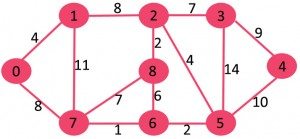该图包含9个顶点（V）和14个边（E）。因此，形成的最小生成树将具有（9 – 1）= 8 个边。

步骤1：每条边按顺序来排序

``` 1     　　/**
2          * 排序后：
3          * 权重-src-dest
4          * 1 6 7
5          * 2 2 8
6          * 2 5 6
7          * 4 0 1
8          * 4 2 5
9          * 6 6 8
10          * 7 2 3
11          * 7 7 8
12          * 8 0 7
13          * 8 1 2
14          * 9 3 4
15          * 10 4 5
16          * 11 1 7
17          * 14 3 5
18          */```

步骤2+步骤3：：利用按权重排好序的边数组，每次选取最小边，并检测是否成环MST不能有环，所以这里涉及一个并查集的概念，并查集是对这个 Kruskal 算法进行优化的。

1）数组中一个接一个地选取所有边取边6-7：不形成循环，将其包括在内。2）选取边2-8：不形成循环，将其包括在内。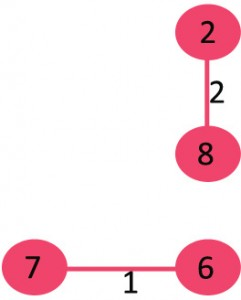3）选取边5-6：不形成循环，将其包括在内。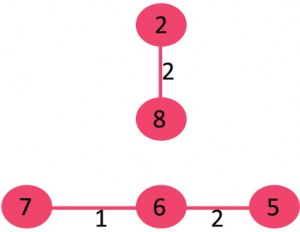4）选取边0-1：不形成循环，将其包括在内。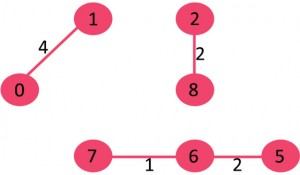5）选取边2-5：不形成循环，将其包括在内。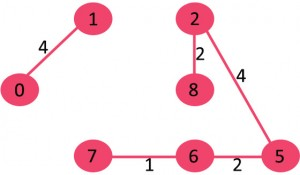6）选取边6-8：由于包括该边会导致成环，因此将其丢弃。

7）选取边2-3：不形成循环，将其包括在内。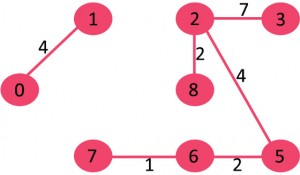8）选取边7-8：由于包括该边会导致循环，因此请将其丢弃。

9）选取边0-7不形成循环，将其包括在内。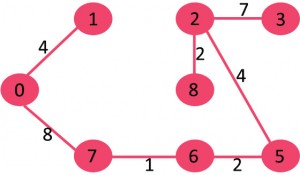10）选取边1-2：由于包括该边会导致循环，因此请将其丢弃。

11）选取边3-4：不形成循环，将其包括在内。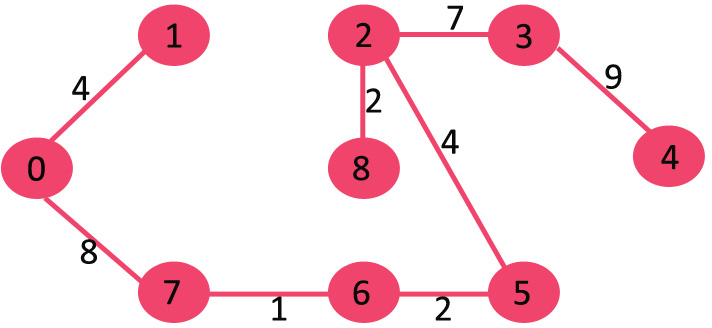由于包含的边数等于（V – 1），因此算法结束。

# 三、算法代码

### 并查集：

在计算机科学中，并查集是一种树型的数据结构，用于处理一些不交集（Disjoint Sets）的合并及查询问题。有一个联合-查找算法union-find algorithm）定义了两个用于此数据结构的操作：

• `Find`：确定元素属于哪一个子集。它可以被用来确定两个元素是否属于同一子集。

• `Union`：将两个子集合并成同一个集合。

并查集树是一种将每一个集合以表示的数据结构，其中每一个节点保存着到它的父节点的引用。

在并查集树中，每个集合的代表即是集合的根节点。“查找”根据其父节点的引用向根行进直到到底树根。“联合”将两棵树合并到一起，这通过将一棵树的根连接到另一棵树的根。实现这样操作的一种方法是：

查找元素 i 的集合，根据其父节点的引用向根行进直到到底树根

```1     private int find(Subset[] subsets, int i) {
2         if (subsets[i].parent != i)
3             subsets[i].parent = find(subsets, subsets[i].parent);   // 路径压缩，找到最久远的祖先时“顺便”把它的子孙直接连接到它上面
4         return subsets[i].parent;
5     }```

将两组不相交集合 x 和 y 进行并集，找到其中一个子集最父亲的父亲（也就是最久远的祖先），将另外一个子集的最久远的祖先的父亲指向它

``` 1     public void union(Subset[] subsets, int x, int y) {
2         int xroot = find(subsets, x);
3         int yroot = find(subsets, y);
4
5         /* 在高秩树的根下附加秩低树（按秩划分合并） */
6         if (subsets[xroot].rank < subsets[yroot].rank) {
7             subsets[xroot].parent = yroot;
8         } else if (subsets[xroot].rank > subsets[yroot].rank){
9             subsets[yroot].parent = xroot;
10         } else {    // 当两棵秩同为r的树联合(作并集)时，它们的秩r+1
11             subsets[yroot].parent = xroot;
12             subsets[xroot].rank++;
13         }
14     }```

同时使用路径压缩、按秩（rank）合并优化的程序每个操作的平均时间仅为 O(α (n))，其中α (n) 是 n=f(x)=A(x, x) 的反函数，A 是急速增加的阿克曼函数。因为 α(n) 是其反函数，故 α (n) 在 n 十分巨大时还是小于 5。因此，平均运行时间是一个极小的常数。实际上，这是渐近最优算法。

### Kruskal算法

使用算法的思想来构造MST。

``` 1     /**
2      * 使用Kruskal算法构造MST
3      */
4     public void kruskalMST() {
5         Edge[] result = new Edge[V]; // 将存储生成的MST
6         int e = 0;                   // 用于result[]的索引变量
7         int i = 0;                   // 用于排序的边缘索引变量
8         for (i = 0; i < V; ++i) {
9             result[i] = new Edge();
10         }
11
12         /* 步骤一：对点到点的边的权重进行排序 */
13         Arrays.sort(edges);
14
15         /* 创建V个子集*/
16         Subset[] subsets = new Subset[V];
17         for (i = 0; i < V; i++) {
18             subsets[i] = new Subset();
19         }
20
21         /* 使用单个元素创建V子集 */
22         for (int v = 0; v < V; v++) {
23             subsets[v].parent = v;
24             subsets[v].rank = 0;    // 单元素的树的秩定义为0
25         }
26
27         /* 用于挑选下一个边的索引 */
28         i = 0;
29
30         while (e < V-1) {
31             /* 步骤2：选取最小的边缘, 并增加下一次迭代的索引 */
32             Edge next_edge = edges[i++];
33
34             int x = find(subsets, next_edge.src);
35             int y = find(subsets, next_edge.dest);
36
37             /* 如果包括此边不引起mst成环（树本无环），则将其包括在结果中并为下一个边增加结果索引存下一条边 */
38             /* 这里判断两个元素是否属于一个子集 */
39             if (x != y) {
40                 result[e++] = next_edge;
41                 union(subsets, x, y);
42             }
43             /* 否则丢弃next_edge */
44         }
45
46         /* 打印result[]的内容以显示里面所构造的MST */
47         System.out.println("Following are the edges in the constructed MST");
48         for (i = 0; i < e; ++i) {
49             System.out.println(result[i].src + " -- " + result[i].dest + " == " + result[i].weight);
50         }
51     }```

平均时间复杂度为O (|E|·log |V|)，其中 E 和 V 分别是图的边集和点集。```  1 package algorithm.mst;
2
3 import java.util.Arrays;
4
5 public class KruskalAlgorithm {
6     /* 顶点数和边数 */
7     private int V, E;
8     /* 所有边的集合 */
9     private Edge[] edges;
10
11     /**
12      * 创建一个V个顶点和E条边的图
13      *
14      * @param v
15      * @param e
16      */
17     public KruskalAlgorithm(int v, int e) {
18         V = v;
19         E = e;
20         edges = new Edge[E];
21         for (int i = 0; i < e; i++) {
22             edges[i] = new Edge();
23         }
24     }
25
26     /**
27      * 查找元素i的集合（路径压缩）
28      * 根据其父节点的引用向根行进直到到底树根
29      *
30      * @param subsets
31      * @param i
32      * @return
33      */
34     private int find(Subset[] subsets, int i) {
35         if (subsets[i].parent != i)
36             subsets[i].parent = find(subsets, subsets[i].parent);   // 路径压缩，找到最久远的祖先时“顺便”把它的子孙直接连接到它上面
37         return subsets[i].parent;
38     }
39
40     /**
41      * 将两组不相交集合x和y进行并集（按秩合并）
42      * 这个方法找到其中一个子集最父亲的父亲（也就是最久远的祖先），将另外一个子集的最久远的祖先的父亲指向它。
43      * <p>
44      *     并查集树的最基础的表示方法，这个方法不会比链表法好，
45      *     这是因为创建的树可能会严重不平衡。
46      *     所以采用“按秩合并”来优化。
47      * </p>
48      * <p>
49      *     即总是将更小的树连接至更大的树上。因为影响运行时间的是树的深度，
50      *     更小的树添加到更深的树的根上将不会增加秩除非它们的秩相同。
51      *     在这个算法中，术语“秩”替代了“深度”，因为同时应用了路径压缩时秩将不会与高度相同。
52      * </p>
53      *
54      * @param subsets
55      * @param x
56      * @param y
57      */
58     public void union(Subset[] subsets, int x, int y) {
59         int xroot = find(subsets, x);
60         int yroot = find(subsets, y);
61
62         /* 在高秩树的根下附加秩低树（按秩划分合并） */
63         if (subsets[xroot].rank < subsets[yroot].rank) {
64             subsets[xroot].parent = yroot;
65         } else if (subsets[xroot].rank > subsets[yroot].rank){
66             subsets[yroot].parent = xroot;
67         } else {    // 当两棵秩同为r的树联合(作并集)时，它们的秩r+1
68             subsets[yroot].parent = xroot;
69             subsets[xroot].rank++;
70         }
71     }
72
73     /**
74      * 使用Kruskal算法构造MST
75      */
76     public void kruskalMST() {
77         Edge[] result = new Edge[V]; // 将存储生成的MST
78         int e = 0;                   // 用于result[]的索引变量
79         int i = 0;                   // 用于排序的边缘索引变量
80         for (i = 0; i < V; ++i) {
81             result[i] = new Edge();
82         }
83
84         /* 步骤一：对点到点的边的权重进行排序 */
85         Arrays.sort(edges);
86
87         /* 创建V个子集*/
88         Subset[] subsets = new Subset[V];
89         for (i = 0; i < V; i++) {
90             subsets[i] = new Subset();
91         }
92
93         /* 使用单个元素创建V子集 */
94         for (int v = 0; v < V; v++) {
95             subsets[v].parent = v;
96             subsets[v].rank = 0;    // 单元素的树的秩定义为0
97         }
98
99         /* 用于挑选下一个边的索引 */
100         i = 0;
101
102         while (e < V-1) {
103             /* 步骤2：选取最小的边缘, 并增加下一次迭代的索引 */
104             Edge next_edge = edges[i++];
105
106             int x = find(subsets, next_edge.src);
107             int y = find(subsets, next_edge.dest);
108
109             /* 如果包括此边不引起mst成环（树本无环），则将其包括在结果中并为下一个边增加结果索引存下一条边 */
110             /* 这里判断两个元素是否属于一个子集 */
111             if (x != y) {
112                 result[e++] = next_edge;
113                 union(subsets, x, y);
114             }
115             /* 否则丢弃next_edge */
116         }
117
118         /* 打印result[]的内容以显示里面所构造的MST */
119         System.out.println("Following are the edges in the constructed MST");
120         for (i = 0; i < e; ++i) {
121             System.out.println(result[i].src + " -- " + result[i].dest + " == " + result[i].weight);
122         }
123     }
124
125     public static void main(String[] args) {
126         /**
127          * 排序后：
128          * 权重-src-dest
129          * 1 6 7
130          * 2 2 8
131          * 2 5 6
132          * 4 0 1
133          * 4 2 5
134          * 6 6 8
135          * 7 2 3
136          * 7 7 8
137          * 8 0 7
138          * 8 1 2
139          * 9 3 4
140          * 10 4 5
141          * 11 1 7
142          * 14 3 5
143          */
144         int V = 9;
145         int E = 14;
146         KruskalAlgorithm graph = new KruskalAlgorithm(V, E);
147
148         /* 另一个用例的图:
149               1 --- 2 --- 3
150             / |     | \   | \
151            0  |     8  \  |  4
152             \ |  /  |   \ | /
153               7 --- 6 --- 5
154          */
155
156         // 添加边 0-1
157         graph.edges.src = 0;
158         graph.edges.dest = 1;
159         graph.edges.weight = 4;
160
161         // 添加边 0-7
162         graph.edges.src = 0;
163         graph.edges.dest = 7;
164         graph.edges.weight = 8;
165
166         // 添加边 1-2
167         graph.edges.src = 1;
168         graph.edges.dest = 2;
169         graph.edges.weight = 8;
170
171         // 添加边 1-7
172         graph.edges.src = 1;
173         graph.edges.dest = 7;
174         graph.edges.weight = 11;
175
176         // 添加边 2-3
177         graph.edges.src = 2;
178         graph.edges.dest = 3;
179         graph.edges.weight = 7;
180
181         // 添加边 2-5
182         graph.edges.src = 2;
183         graph.edges.dest = 5;
184         graph.edges.weight = 4;
185
186         // 添加边 2-8
187         graph.edges.src = 2;
188         graph.edges.dest = 8;
189         graph.edges.weight = 2;
190
191         // 添加边 3-4
192         graph.edges.src = 3;
193         graph.edges.dest = 4;
194         graph.edges.weight = 9;
195
196         // 添加边 3-5
197         graph.edges.src = 3;
198         graph.edges.dest = 5;
199         graph.edges.weight = 14;
200
201         // 添加边 4-5
202         graph.edges.src = 4;
203         graph.edges.dest = 5;
204         graph.edges.weight = 10;
205
206         // 添加边 5-6
207         graph.edges.src = 5;
208         graph.edges.dest = 6;
209         graph.edges.weight = 2;
210
211         // 添加边 6-7
212         graph.edges.src = 6;
213         graph.edges.dest = 7;
214         graph.edges.weight = 1;
215
216         // 添加边 6-8
217         graph.edges.src = 6;
218         graph.edges.dest = 8;
219         graph.edges.weight = 6;
220
221         // 添加边 7-8
222         graph.edges.src = 7;
223         graph.edges.dest = 8;
224         graph.edges.weight = 7;
225
226         graph.kruskalMST();
227
228         /* 用例通过算法得出的MST如下:
229                1    2 -- 3
230              /      | \   \
231             0       8  \   4
232              \          \
233                7 -- 6 -- 5
234          */
235     }
236
237     /**
240      */
241     class Edge implements Comparable<Edge> {
242         /* 这条边的两个顶点和它的权重 */
243         private int src, dest, weight;
244
245         @Override
246         public int compareTo(Edge o) {
247             return this.weight - o.weight;
248         }
249     }
250
251     /**
252      * 联合查找子集的类
253      */
254     class Subset {
255         /* 其祖先和秩 */
256         private int parent, rank;
257     }
258 }```
View Code